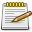# Matrix

Jump to: navigation, searchScript Example Missing Function Matrix needs a script example, help out by writing one. Before submitting check out Editing Guidelines Script Examples.

Matrices are one of the most powerful features of MTA OOP. We did have a presence of Matrices before with getElementMatrix, but we were given an ugly disgusting table to play with. Now, with the new Matrix class, we can make and magically manipulate Matrices.

## Methods

### create

This is default constructor for the Matrix class and returns a Matrix object. You can instantiate a Matrix object in several ways, as described below.

`matrix Matrix ( Vector3 position[, Vector3 rotation] )`
• position: The position vector of the matrix
• rotation: The rotation vector of the matrix

`matrix Matrix ( Matrix matrixToClone )`
• matrixToClone: A matrix you want to make a clone of

`matrix Matrix()`
• You can call this method without parameters to initialize a zero matrix

### transformPosition

This method transforms a given position vector using the Matrix.

`Vector3 Matrix:transformPosition ( Vector3 position )`
• position: The position vector you want to transform

#### Example

This example teleports a random player to a location 5 meters in front of him

```local player = getRandomPlayer()
local desiredRelativePosition = Vector3(0, 5, 0) -- 5 meters front of player is a y = 5 vector
local matrix = player.matrix
local newPosition = matrix:transformPosition(desiredRelativePosition)
player.position = newPosition
```

### getPosition

This method returns the position vector of a given matrix.

`Vector3 Matrix:getPosition()`

#### Example

This example prints the position of a random player.

```local player = getRandomPlayer()
local matrix = player.matrix
local position = matrix:getPosition()
outputChatBox("x: " .. position:getX() .. ", y: " .. position:getY() .. ", z:" .. position:getZ())
```

### getRotation

This method returns the rotation vector of a given matrix.

`Vector3 Matrix:getRotation()`

#### Example

This example prints the rotation of a random player.

```local player = getRandomPlayer()
local matrix = player.matrix
local rotation = matrix:getRotation()
outputChatBox("rx: " .. rotation:getX() .. ", ry: " .. rotation:getY() .. ", rz:" .. rotation:getZ())

```

## Using Matrices

Say you wanted to create a bin - object 1337 - two units in front of a player. You don't want to manually do trigonometry and you don't want to play with the complicated tables. You just want to get the position two units in front of the player whilst taking into account the rotation of the player. You only need to use Matrix.getForward, Matrix.getPosition and getElementMatrix. It's just:

```Object ( 1337, player.matrix.position + player.matrix.forward * 2 )
```

If you wanted the opposite of matrix.forward you'll still use matrix.forward but you minus it instead of matrix.backward which doesn't exist. Same applies with matrix.right and matrix.up. To make the bin object appear behind a player:

```Object ( 1337, player.matrix.position - player.matrix.forward * 2 )
```

## Why not stick to the good ol' tables?

Say you'd like to get find the position underneath a vehicle. This is the position at any rotation. So if it was upside down, the Z value would be higher than the vehicle Z value. If the vehicle was the right way round, the Z value for underneath car would be less than the Z value for the car.

```--
-- Instead of:
--
local matrix = getElementMatrix(vehicle)
local offX = 0 * matrix + 0 * matrix - 1 * matrix + matrix
local offY = 0 * matrix + 0 * matrix - 1 * matrix + matrix
local offZ = 0 * matrix + 0 * matrix - 1 * matrix + matrix

local pX, pY, pZ = getElementPosition(vehicle)
local positionBelow = {offX-pX, offY-pY, offZ-pZ}

--
-- You only have to do:
--
local positionBelow = vehicle.position - vehicle.matrix.up
```